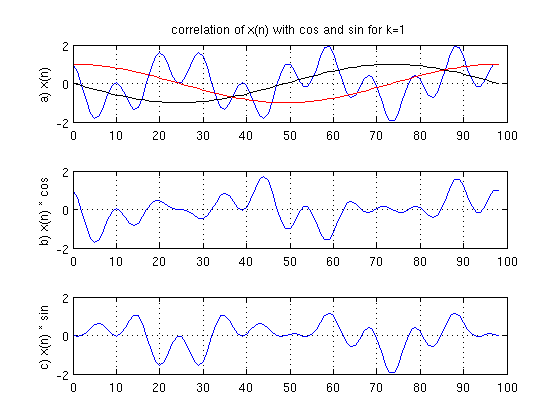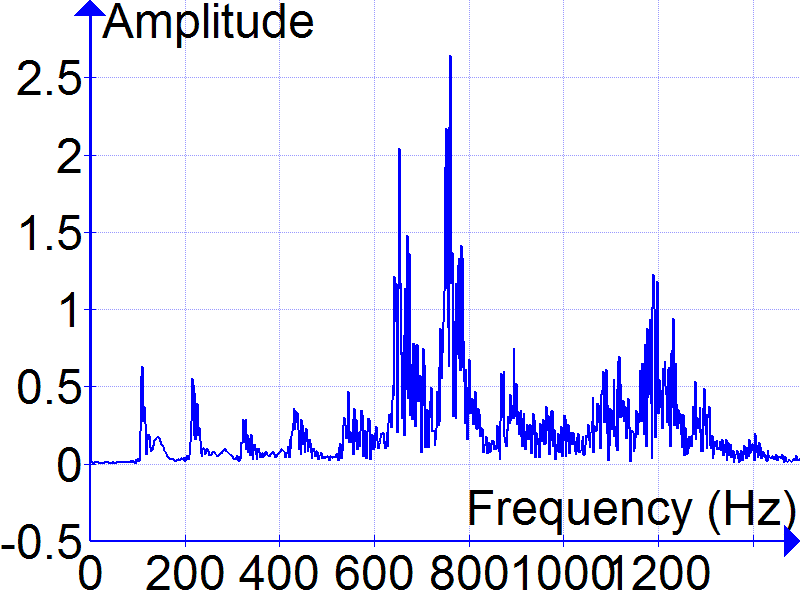# Some questions about Fourier transformation

Richard_Steele
Hi,
I'm writting a program in the computer and I've to perform a fast Fourier transform to get the frequency domain information. I've read different website, I've watched some videos, etc and I don't fully understand the whole theory about FFT. I've to say that I don't have a solid mathematics and physics background.

As far I know the FFT is an algorithm to represent the information in the frequency domain. The audio signal I've is in the time domain. The purpouse of the FFT is to obtain the main 'ingredients' and their quantities from the original time domain signal. It's like if I've an ice-cream and I've to obtain the ingredients (3 parts of sugar, 5 parts of milk...).

To obtain the frequency information from the time domain signal, I've to apply filters and observe the results. These filters are harmonic frequencies, one at time. So I've to compare, one by one, the basis signals (harmonics) with the time domain signal to see how much correlation there is.

Each harmonic has to be compared in two ways: comparing the real part (cosine) and comparing the imaginary part (sine) of that harmonic with the time domain signal. A high correlation means that when the time domain signal is positive, the harmonic is also positive and when it's negative the harmonic is also negative. The cosine correlation has a result a real number. The sine correlation has a result an imaginary number. The real + imaginary number is a complex number. Well, the absolute value of that complex number in a given point, is the magnitude of the specific spectrum. All this is right?

Time domain signal
Cosine
SineOne of the parts I don't understand is after performing the correlation. The result is the above graph and I've to obtain a number from that graph. How can I obtain the correlation value using that graphic? For example, if I've to obtain the sine correlation number, how can I get that number from the graphic?

Gold Member
I'm pretty good at Fourier transforms, and I can't tell what you are trying to do.

Here's a paper that explains some things

http://arxiv.org/ftp/physics/papers/0605/0605154.pdf

You could also post your data (amplitude vs. time) and let us run a Fourier transform that we know is accurate. (Or you could download our code from the link in my signature.)

In general, Fourier transform code should be validated either with known waveforms (that give predictable results) or in concert with known good code to confirm that the new code and the known good code give the same results.

Audacity has built in FFT code for doing quick analysis of sound waveforms.

Last edited:
•Richard_Steele
Richard_Steele
I'm pretty good at Fourier transforms, and I can't tell what you are trying to do.

Here's a paper that explains some things

http://arxiv.org/ftp/physics/papers/0605/0605154.pdf

You could also post your data (amplitude vs. time) and let us run a Fourier transform that we know is accurate.

In general, Fourier transform code should be validated either with known waveforms (that give predictable results) or in concert with known good code to confirm that the new code and the known good code give the same results.

Audacity has built in FFT code for doing quick analysis of sound waveforms.
Well, all the information I've written here is from different websites and videos I was watching.

I can post the information here, no problem. It's 8bit mono audio information, so each byte represents a point in the time domain representation. Values go from 0 to 255. Is that ok to validate with your software?

Gold Member
Our software is written to read text files. There are just too many binary formats to support them all.

Richard_Steele
Our software is written to read text files. There are just too many binary formats to support them all.
Ok, so I will write a program to extract the information and write it in a text file. It will take time because I'm not very good with programming.

Richard_Steele
Our software is written to read text files. There are just too many binary formats to support them all.
Here you've as an attached txt file.

#### Attachments

• audio 8bit mono.txt
128.5 KB · Views: 294
Gold Member
What is the sample rate (or time step)?

Gold Member
I had to assume a sample rate of 44100 samples per second to meaningfully compute the FFT in sensible units (frequency in Hz).

The graph is shown below. If my guess at the sample rate is right, the sound is a musical A note with a fundamental frequency of 110 Hz and lots of overtones, possibly due to distortion, possibly due to an instrument with lots of overtones. The output fft is also attached as a text file (amplitude vs. frequency). The third column in the file is phase (in radians).

There is a strong temptation in the fft world to neglect the independent variable. This is a dangerous practice when interpreting the physical meaning of Fourier transforms. When you get your fft code written properly, your output should look the same, give or take a multiplicative constant for the amplitude as well as a possible horizontal stretch if I guessed wrong at the sample rate. Note that the fft returns data out to a frequency of 0.5 times the sample rate. The outout data is there, but there is little in terms of meaningful features.#### Attachments

• Test.txt
381.1 KB · Views: 335
•Richard_Steele
Richard_Steele
What is the sample rate (or time step)?
Sorry, the sample rate is 44100 Hz

Richard_Steele
I had to assume a sample rate of 44100 samples per second to meaningfully compute the FFT in sensible units (frequency in Hz).

The graph is shown below. If my guess at the sample rate is right, the sound is a musical A note with a fundamental frequency of 110 Hz and lots of overtones, possibly due to distortion, possibly due to an instrument with lots of overtones. The output fft is also attached as a text file (amplitude vs. frequency). The third column in the file is phase (in radians).

There is a strong temptation in the fft world to neglect the independent variable. This is a dangerous practice when interpreting the physical meaning of Fourier transforms. When you get your fft code written properly, your output should look the same, give or take a multiplicative constant for the amplitude as well as a possible horizontal stretch if I guessed wrong at the sample rate. Note that the fft returns data out to a frequency of 0.5 times the sample rate. The outout data is there, but there is little in terms of meaningful features.
Yes, the same rate is 44100 samples per second (sorry about the reply. I wasn't connected). The sound is human voice saying the letter 'A' all the time. Thanks for the text file.

I'm reading carefully the pdf you post yesterday and also looking at the text file that contain the results.

I will reply you after I understand it a little bit better. It will take time (around 1 day). Thanks again.

Richard_Steele
...
I've examined the original wav file in Audacity and I get the same results as your graphic.

With the program I'm trying to write, I've to understand more technical details. I've read your pdf but I think the part I don't understand is a little bit more technical.

Well, imagine that I've 512 samples to analyze from an audio signal (8bit, 1 channel, 44.100 samples/sec) and I want to get the information in the frequency domain, from 1 Hz to 200 Hz. The first step the program has to do is to calculate the real (cosine) and the imaginary (sine) part from the audio signal.
I've seen a source code about DFT and I think FFT could be similar. Well, we've 512 samples and 200 basic sinusoidal signals to correlate. So I've to have a matrix of 200 variables that contains each basic harmonic (first harmonic ... 200th).

Calculations:
Real part of the first frequency: Real part value = real part value + value of the first sample * Cosine (2 * PHI * (cycles per second of the first frequency in this case = 1) * index of the sample in the time domain * number of samples to analyze (in this case = 512)

I won't keep writting because I don't know if this is correct or not.
Is this ok?

Gold Member
If you just do the complex Fourier transform (like the output of the fft I posted or using the software from my link), then this information contains both the cos() and the sin() part as subsets. The cos() part is simply the real part at each frequency. The sin() part is the imaginary part.

Richard_Steele
If you just do the complex Fourier transform (like the output of the fft I posted or using the software from my link), then this information contains both the cos() and the sin() part as subsets. The cos() part is simply the real part at each frequency. The sin() part is the imaginary part.
I ask you those questions because I want to write a code to get a graphic like yours and I've to understand more technical details about fft.
As far I know to get the magnitude of a specific frequency (for example, the magnitude of 500 Hz in the frequency domain) is needed to get the absolute value of the complex number (real part result + imaginary part result). Is thi right?